# Hardest colleges to get into in South Dakota

Top 9 colleges in South Dakota with the lowest acceptance rates
What are the hardest colleges to get into in South Dakota? We've got you covered. We've compiled a national college database and have created a list of the hardest universities to get into in South Dakota below. These are the hardest 4 year colleges to get into in South Dakota, and sometimes some of the hardest colleges to get into in the US. You could even say these are the best colleges in South Dakota. We also include each college's average SAT and ACT scores so that you can see where you're most competitive. Read on to find out more.

## Mount Marty College acceptance rate

Mount Marty College acceptance rate is 69.2%.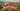The average SAT score for Mount Marty College is 1110.

• SAT composite: 1110
• SAT math: 555

The average ACT score for Mount Marty College is 21.

## Dakota Wesleyan University acceptance rate

Dakota Wesleyan University acceptance rate is 73.3%.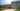The average SAT score for Dakota Wesleyan University is 1080.

• SAT composite: 1080
• SAT math: 540

The average ACT score for Dakota Wesleyan University is 22.

## Dakota State University acceptance rate

Dakota State University acceptance rate is 83.2%.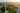The average SAT score for Dakota State University is 1090.

• SAT composite: 1090
• SAT math: 550

The average ACT score for Dakota State University is 23.

## South Dakota School of Mines and Technology acceptance rate

South Dakota School of Mines and Technology acceptance rate is 84.5%.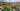The average SAT score for South Dakota School of Mines and Technology is 1220.

• SAT composite: 1220
• SAT math: 650

The average ACT score for South Dakota School of Mines and Technology is 27.

## University of South Dakota acceptance rate

University of South Dakota acceptance rate is 85.8%.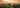The average SAT score for University of South Dakota is 1151.

• SAT composite: 1151
• SAT math: 580

The average ACT score for University of South Dakota is 23.

## Black Hills State University acceptance rate

Black Hills State University acceptance rate is 87%.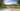The average SAT score for Black Hills State University is 1080.

• SAT composite: 1080
• SAT math: 540

The average ACT score for Black Hills State University is 21.

## Northern State University acceptance rate

Northern State University acceptance rate is 88.4%.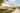The average SAT score for Northern State University is 1098.

• SAT composite: 1098
• SAT math: 570

The average ACT score for Northern State University is 21.

## South Dakota State University acceptance rate

South Dakota State University acceptance rate is 90.8%.The average SAT score for South Dakota State University is 1110.

• SAT composite: 1110
• SAT math: 570

The average ACT score for South Dakota State University is 23.

## University of Sioux Falls acceptance rate

University of Sioux Falls acceptance rate is 91.3%.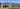The average SAT score for University of Sioux Falls is 1108.

• SAT composite: 1108
• SAT math: 550